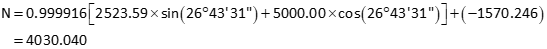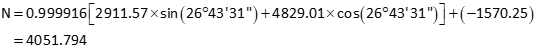### 5. Numeric Example

A crew surveyed the exterior of a parcel, Figure J-23. They assumed the coordinates of the southernmost corner (1001) and used the record bearing for the southwesterly line (1001-1002)Figure J-23
Exterior survey

 Point North East 1001 3684.22 2208.05 1002 4030.04 1940.56 1003 4244.27 2366.04 1004 3802.06 2607.77

Later, a second survey crew was sent out to locate the existing drainfield. They did not have the first crew's coordinates so they occupied the northernmost corner (NE), for which they assumed coordinates, and backsighted the westernmost corner (NW), for which they assumed a direction. They then shot the drainfield corners. As a check, they also shot the distance from NE to NW. Figure 24 is a summary of their survey.Figure J-24
Drainfield survey

 Point North East NE 5000.00 3000.00 501 4914.03 2776.03 502 4814.98 2786.38 503 4829.01 2911.57 504 4934.06 2895.69

Assumed dir NE-NW: N 90°00'00" W
Distance NE-NW: 476.41

The data was combined in  a CAD drawing with the result shown in Figure J-25.Figure J-25 Combined field data

Using the boundary survey as the reference system, translate the drainfield data.

#### a. Determine transformation parameters

Step (1) Scale

Length of line NE-NW is 476.41', line 1003-1002 is 476.37'.

From Equation J-1:This represents a distortion of 1/(1-0.999916) = 1/11,900. If this is within tolerance, then the scale factor can be ignored. We will apply it in this example.

Step (1) Rotation angle

Line NE-NW coincides with line 1003-1002; Convert the bearings to azimuths and compute the rotation angle.

 Line NE-NWLine 1003-1002Using Equation J-2-

Step (3) Translations

Use the coordinates of points 1003 and NE in Equations J-3 and J-4 to determine the translations.Step (4) Math check

For a parameters math check, convert NW to see if it matches the coordinates of 1002.

In the From system, NW's coordinates are:Transform and check:Yuo, it checks.

#### b. Using Equations J-5 and J-6 transform the drainfield corners: 501-504

Point 501Point 502Point 503Point 504